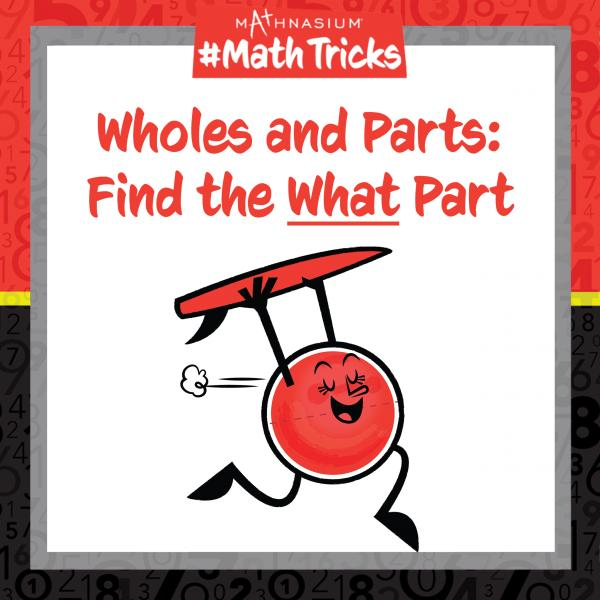877-601-6284
Get Started by Finding a Local Centre

# Mathnasium #MathTricks: Fractions (Wholes and Parts Part 3)

Aug 23, 2023Welcome to Mathnasium’s Math Tricks series. Today we are finding the value of the what part, in a Wholes and Parts problem.

In a Wholes and Parts problem, sometimes we have the whole and the given part, and we need to find the what part. When we are asked, “What part of a bigger number is a smaller number?” it is the same as being asked, “What fraction of the bigger number is the smaller number?” — this gives us the what part. Note that here, “is” means “equals.”

To find the value of the what part, we use the following steps:

1. Identify the whole and the given part.
2. Compare the given part to the whole as a fraction in lowest terms.

Follow the examples below to find the what part.

##### Example 1: 9 is what part of 54?

Step 1: Identify the whole and the given part.
The whole is 54.
The given part is 9.

Step 2: Compare the given part to the whole as a fraction in lowest terms.

9/54 = 1/6

##### Example 2: A candy jar contains 9 chocolates, 14 gumballs, and 7 mints. What part of the candies are chocolates?

Step 1: Identify the whole and the given part.
The whole is 30 (9 chocolates + 14 gumballs + 7 mints).
The given part is 9 chocolates.

Step 2: Compare the given part to the whole as a fraction in lowest terms.

9/30 = 3/10

Now, with this strategy, you are ready to use this Mathnasium Math Trick to find the value of the what part in a Wholes and Parts problem. Click here for more practice problems, then check your answers here.

If you missed this, or any of our other Math Tricks videos, check them out on our YouTube channel!

Do you have a math trick you want to see? Submit your request at: http://bit.ly/MathnasiumMathTricks.

## SEE HOW MATHNASIUM WORKS FOR YOUR SITUATION

### My Child is:## OUR METHOD WORKS

Mathnasium meets your child where they are and helps them with the customized program they need, for any level of mathematics.Early LearnersElementary SchoolMiddle SchoolHigh School